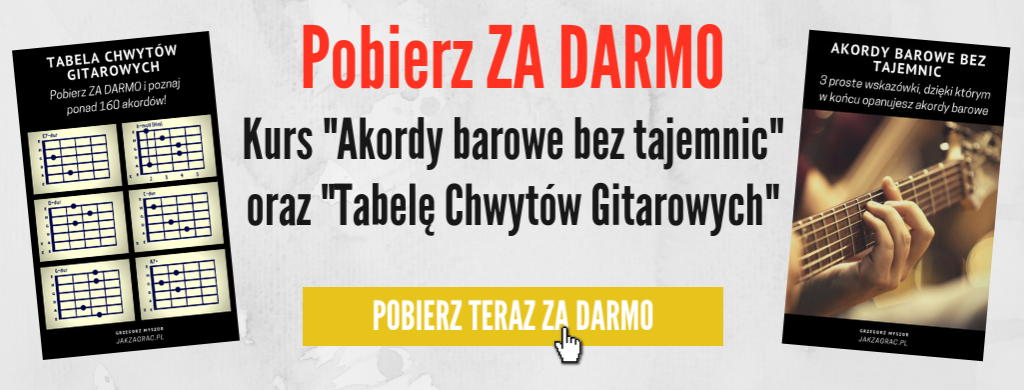# Jak zagrać na gitarze Let it be – The Beatles – Chwyty i tekst

## Let it be – The Beatles

*Pobierz tekst z chwytami w PDF

C                     G
1. When I find myself in times of trouble
a                   F
Mother Mary comes to me
C                            G
Speaking words of wisdom
F  C d C
Let it be
C                          G
And in my hour of darkness
a                         F
She is standing right in front of me,
C                            G
Speaking words of wisdom
F  C d C
Let it be

a           G            F           C
Ref. Let it be, let it be, let it be, let it be,
C                          G                   F   C d C
Whisper words of wisdom, let it be.

C                         G
2. And when the broken hearted people
a                   F
Living in the world agree
C                        G
F  C d C
Let it be
C                              G
For though they may be parted there is
a                           F
Still a chance that they will see
C                        G
F  C d C
Let it be

a           G            F           C
Ref. Let it be, let it be, let it be, let it be,
C                      G                   F   C d C
Yeah, there will be an answer, let it be

a           G            F           C
{Let it be, let it be, let it be, let it be,
C                          G                   F   C d C
Whisper words of wisdom, let it be.} x2

C                          G
3. And when the night is cloudy
a                     F
There is still a light that shines on me
C                       G
Shine on until tomorrow
F  C d C
Let it be
C                     G
I wake up to the sound of music
a                    F
Mother Mary comes to me
C                            G
Speaking words of wisdom
F  C d C
Let it be

a           G            F           C
Ref. Let it be, let it be, let it be, let it be,
C                      G                   F   C d C
Yeah, there will be an answer, let it be

a           G            F           C
{Let it be, let it be, let it be, let it be,
C                          G                   F   C d C
Whisper words of wisdom, let it be.} x2